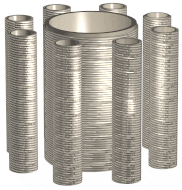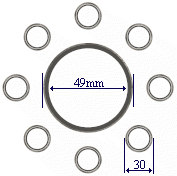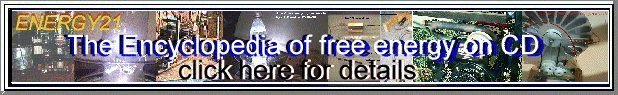#### Hubbard Matrix### Introduction.

For those new to the area of matrix mathematics the following is an evaluation of Alfred Hubbards nine(9) coil alternative energy generator. The author G.D.Mutch has reviewed one design of Alfred Hubbards material an arrived at the conclusion that Hubbards technology can be applied to matrix mathematics, which in part is very similar to Professor J.R.R.Searls 'Law of the Squares ©'. The following material will show people how Hubbards design is first formulated into a balanced mathematical square matrix and then transformed out of the matrix into mathematical ratios that can be used in an actual working model...

When we talk about energy you may like to develop a mental picture that all atoms are whirling matrices of aetheric energy. You then simply have to understand that putting different density or volumes of materials together you can utilise the sum difference of these two densities/volumes to multiple or increase an input energy packet of the correct frequency. The matrix number 6 is an interesting number in that it is mostly a none sharing number which can be useful in energy multiplying matrices. A good example of this is the golden section or fibonacci sequence 0.6 or 1.6. The value 1.6 is a very popular value and shows up in numerous everyday calculations. Six(6) sided cubic crystal structures of elements appear to make the best combinations for energy utilisation. It is my belief that the none sharing property of six sided crystals cause non harmonic energy packets coming into their atomic matrix/orbits to multiply the aetheric spin of this input energy. The atomic structure then rejects the arriving input energy packet out of the crystals atomic orbits while trying to rebalance their own energy frame. This then causes the original energy packet to step up from is original quanta an be expelled onto the next crystal. The next crystal then repeats the energy step up process until your reach the final outer layer of the material. To get this multiplying affect you should have at least two or more different materials or substances combined. You should have at lease two different densities or volumes of the same substances. In affect you must have an unbalanced state to get a potential energy output... Professor Searl also uses six sided crystal structures in applications with in the SEG technology. Professor Searl uses a slightly different mathematical matrix process for the SEG calculations to those stated in the following text...

### Hubbards Original Design

Hubbards dimensions on the nine(9) coil transformer design:Top viewSide view

figure 1a

Hubbard Design Outer Inner Total
No. Coils 8 1 9
Diameter mm 30 49
Height mm 146 146
Hubbards Frequencies
5.340 Hz = 2.8 Ghz/ (2^19)
10.681 Hz = 2.8 Ghz/ (2^18)
21.362 Hz = 2.8 Ghz/ (2^17)

figure 1b.

The dimensions in the table of figure 1b are taken from Hubbards actual nine(9) coil design.
Hubbard used a multiply ratio of 5.75 formulated from his knowledge of the Golden Section. Example 49/30 = 1.6333. Hubbard stated that his nine(9) coil design above stepped up the output power compared to the input power by a ratio of 3:1.

### Matrix Formulation.

The author will transform the values of Hubbards nine(9) coil design of figure1 into a 3 x 3 balanced matrix. The 8 Coils around 1 inner coil equates to 9 coils which can be transformed into the 3 x 3 matrix below (see figure 2).

 63.25 30 53.75 39.5 49 58.5 44.25 68 34.75

Matrix = 147

Figure 2.

Matrix Values Centre Outer * 3rd.
No. Coils 1 8 1
Diameter mm 49 30 68
Height mm 147 147 147

* Hypothetical optional 3rd Coil.

Figure 3.

#### 1. Derived values from the above matrix :

The above matrix of figure 2 has a sum line value of 147. No matter which way you add the rows, columns or diagonals the line value will add to 147. The following values tabulated in random order are then derived from the balanced matrix . These tabulated values are the actual physically wound coil dimensions from which you can build the Hubbard nine(9) coil energy transformer device.

1. 1 x Centre Coil = 49mm Dia
2. 8 x Outer Coils = 30mm Dia.
3. *1 x Hypothetical 3rd coil = 68mm Dia.
4. Cylinder Coil length = 147mm
5. Matrix Step value = 4.75
6. Corners : Centre x 4 = 196
7. 1st Ring : Centre x 8 = 392 (8 cells around the centre.)
8. Matrix Frequency = 441hz

{* This is a hypothetical coil added here by the author. See below text for more information.}

#### 2. Deriving Matrix Coil Turns = Volume :

To derive the turns ratio you simply multiple the centre value 49 by multiples of 2. When you reach a value of Centre x 8 = 392 you will notice that the sum of the 8 outer cells around the centre value also equal the value 392. This is similar to Professor Searls Law of the Squares. See the table of figure 4.

Centre [ 49] Turns Notes
Centre x 4 196 Hubbards original calculation
Centre x 6 294 Hubbards original calculation
Centre x 8 392 * Alternative calculation
Centre x 10 490 * Alternative calculation
Centre x 12 588 Hubbards original calculation
Centre x 14 686 * Alternative calculation
Centre x 16 784 * Alternative calculation

figure 4

(* Denotes alternative calculations that could be used in actual coil designs.)

NB. Alfred Hubbard is obviously using binary in his calculations...

#### 3. Deriving Matrix Copper Wire dimensions.

To derive the copper wire gauge from the matrix you simply divide the turns (see figure 4) by the line value/cylinder length 147mm. Example, 147/196 = 0.75 mm wire diameter. Knowing this last formula we can now construct a table with multiple choice coil dimensions for any derived wire size. (see figure 5)

#### 4. Matrix Final Physical Coil Dimensions :

By using the matrix of figure 2 we have derived the optimal mathematical ratio values from which we can design the actual coil dimensions. Recapping, from the matrix we have derived :

1. Cylinder Diameter
2. Cylinder Length
3. Turns Per Coil/Cylinder Ratio
4. Wire Gauge/Diameter Ratio

We may therefore tabulate all the values in the above steps into one easy to read table.(See figure 5) You may now use this table as a quick reference to build any coil from your chosen wire size.

#### Coil Dimensions Table

Wire size mm 0.186 0.214 0.25 0.3 0.375 0.5 0.75
Turns per 1 cylinder 788 686 588 490 392 294 196
Length @ 30mm dia. 74.267m 64.653m 55.417m 46.181m 36.945m 27.708m 18.472m
Length @ 49mm dia. 121.303m 105.601m 90.515m 75.429m 60.343m 45.257m 30.171m
*Length @ 68mm dia. 168.339m 146.549m 125.613m 104677m 83.742m 62.806m 41.871m

figure 5.

* Denotes the optional 3rd coil dimension. As I am lead to believe, this third coil is not in Hubbards original nine(9) coil design. The author has added this coil here to allow research into its possible further use as a power multiplying coil. It may be obvious to people that using a thicker wire diameter you will lose a corresponding number of turns per coil/cylinder. This then equates to a drop in voltage, but you should also gain with a corresponding current increase. Inversely, if you choose a thinner diameter wire gauge you will have a voltage increase for the corresponding drop in current. The trick would be to choose a wire diameter ratio for the frequency, current and voltage to wish to use.

Hubbard stated he could use copper wire of different diameter/gauges to complete one totally wound coil. The lengths shown in the above table equals the total copper wire length in meters that you will require to wind one complete coil onto the cylinder/former. The values do not include external connection flying leads.You will need extra wire for termination.

To use the above table of figure 5 simply read down the vertical column of the desired wire diameter/gauge, then read off the Turns and the Total Length of wire for each of the 30mm,49mm and 68mm diameter coils. Example, if you chose to use a wire gauge of 0.75mm you would use the following values :

• Wire Gauge : 0.75mm
• Turns : 196
• 30mm : 18.472 m
• 49mm : 30.171 m
• 68mm : 41.871 m ( Optional 3rd coil. )

The values listed above represent one totally wound coil per cylinder. Hubbard used 8 coils on the outer peripheral of the inner coil, so you will need to multiply the 30mm dia values by 8 to get the total resource material required.

END.

### Miscellaneous Errata :

The below original Hubbard frequency information of figure 6 is take from one design of the Hubbards transformer device. The original reference image of Hubbards transformer device was taken from Geoff Egels Hubbard webpage web site. I had once believed, and have now partly confirmed, that Hubbards transformer device follows one of the aetheric/magnetic field matrices which are similar in part to that of J.R.R.Searls 'Law Of The Squares'. I believe the Hubbard coil/transformer device is tuned to the universal energy fields by utilising natural mathematical matrix law ratios.

Simulating Hubbards Math Calculations:

Where and how does Hubbard derived most of his calculations? For example, his step value 5.75.? A suggestion is to use Hubbards coil length 146 / 5.75 = 25.39. This value 25.39 is very close to the value 25.4 which equals the imperial inch to metric value, or maybe binary (2^8) -2 = 254. Why did Hubbard choose the golden section as his start ratio ? Could Hubbard have use this value 1.6 as his base unit of measure ? Other calculations deriving Hubbards original math:

• 49 / 30 = 1.6 (Golden section)
• 1.61 / 0.28 = 5.75 (Step value)
• 0.28 x 2^19 = 146,800.64 (Coil Length ?)
• 146.9 /49 = 2.997959184 ( C in physics )
• 146/5.75 = 25.4 (Imperial inch conversion)
• 0.28 / 2^19 = 0.000000534 (Hubbards freq.)
• 0.28 / 2^18 = 0.00000010681 (Hubbards freq.)
• 0.28 / 2^17 = 0.00000021362 (Hubbards freq.)

Reversing Hubbards calculations we can derive a more accurate value for Hubbards Natural Frequency : 2^19 x 5.340 = 2799697.92 Hz. The following are Hubbards original values along with the authors modified values in the table of figure 6.

Hubbards Original Freq. 2.8 Hubbards Modified Freq. 2.799
5.340 Hz = 2.8 Ghz/ (2^19) 2799697.92 / (2^19) = 5.34 Hz
10.681 Hz = 2.8 Ghz/ (2^18) 2799697.92 / (2^18) = 10.68 Hz
21.362 Hz = 2.8 Ghz/ (2^17) 2799697.92 / (2^17) = 21.36 Hz

figure 6

Hubbard used a coil/cylinder height of 146mm. The true coil/cylinder length ratio according to Hubbards text, should have been his own given ratio 5.75 x 25.4 = 146.05mm. The true line value of 147 mapped from with in the matrix of figure 2 is not the same as Hubbards value 146.05, which is missing 0.95mm. If you take small liberties an assume Hubbards own calculations of 1.44Ghz ( 1/ 0.694444 similar to Bruce Cathies reciprocal harmonic speed of light) as a base ratio; the step value becomes 2.88 ( Hubbards frequency )Ghz /2 = 1.44 Ghz x 3.141592654(pi) = 4.523893421. If you use this 4.52 value as Hubbards step ratio in a new matrix, then the completed matrix line and coil length value becomes 146.97mm. This value is then 0.03 different to the authors 147mm matrix line value of figure 2.

By transposing the matrix step value of figure 2 as Hubbards step value an original frequency values in figure 6, I am able to reverse engineer and derive a value of 4.75 / pi = 1.51 x 2 = 3.02 Ghz. What could this value represent ? I wonder why Hubbard chose binary harmonic values ? Is Hubbard using the values from the table of figure 6 as input an output frequencies ? Example, should you input 2^19 = 524288 hz and the output = 5.340Hz ? Could a lanthanide element series or a radioactive isotope be used as a cylinder former for an input constant pulse frequency, and thus producing an over unity constant output by not having to input an EMF into the primary coil(s) ?

Again using similar math techniques as Hubbard I can simulate his frequency values together with my own values from the matrix of figure 2, and derive the below theoretical comparison table of figure 6. The following table will show the reader how the author derived a constant frequency from a binary ratio and the matrix line value or coil length in this case.

Matrix Values Alternative Hubbard Values
147 (line value ) / 2 ^19 = 0.00028038 146 (line value ) / 2^19 = 0.000278472
2803800 / 2^19 = 5.34782 hz 2784720 / 2^19 = 5.3114
2803800 / 2^18 = 10.6956 hz 2784720 / 2^18 = 10.622
2803800 / 2^17 = 21.3912 hz 2784720 / 2^17 = 21.215

figure 7

Natural Matrix Frequency = 441 Hz
441 Hz = 2.8901376(nearest) Ghz /(2^16) (Derived using Hubbards method)

In my honest opinion I believe Hubbard could have placed another coil over the inner most coil an produced more output power yet again. Hubbard stated that power output of his nine(9) coil design was 3:1 ie. 3 times the output as per the input. I believe if Hubbard used a 3rd coil design as in the matrix above, then the output could step up to 14 :1. The optional 3rd coil could be the 68mm diameter coil from the matrix. Was/is the original coil and cylinder in the original plan design? I am unable to determine if this is the case as I have only perused one portion of Hubbards original designs.

The above miscellaneous errata is just an evaluation of Hubbards math methods which the author has used to simulate possible comparisons against the natural method of a matrix. A couple of different slants on the same math methods have been added above for the reader to compare and research. I feel the reader should first research with Hubbards own successful coil/transformer calculations and design before moving on to the information presented here in. The author presents the above matrix information as a further refinement to Hubbards own methods, to support Hubbards claim to a successful alternative energy device.

G.D. Mutch
Rockhampton .Qld. Australia.
Email : pagemaster@rocknet.net.au

More importantly : don't let ego and greed cloud your better judgement. Please share all your research findings with others. Let's all make a conscious decision to create a cleaner, freer living world for all...

Disclaimer :
The author G.D.Mutch makes no claim as to the correctness and functional use of the matrix with in the application of the Hubbard transformer device. The above information is offered as experimental information only. The user or constructor accepts all responsibility with the use or inability to use the above information. The Matrix Math when applied to the Hubbard coil/transformer device is Copyright © 2000 by G.D.Mutch. You may use the above matrix information as you so wish, provide you acknowledge all credits to Alfred Hubbard, J.R.R.Searl, Bruce Cathie, Geoff Egel and the author G.D.Mutch.

#### Acknowledgments:

The original Hubbard transformer design is Copyright© property of Alfred Hubbard 1929...

Geoff Egels Natural Energy Web Site
'Law Of The Squares Books' are Copyright of Prof. J.R.R.Searl.
Bruce Cathie Book Series: Harmonic Universe